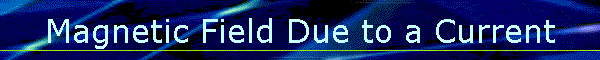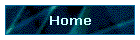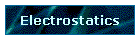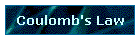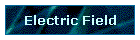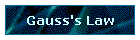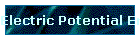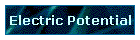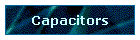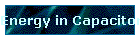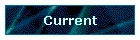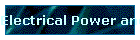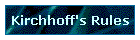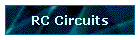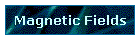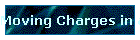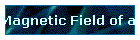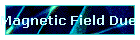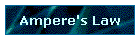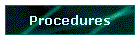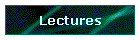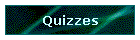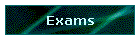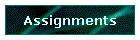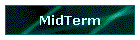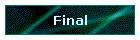Phys 4, Section 2                                                         Feb 22, 2002     Magnetic Field of a Current Element   Amount of charge dQ in a small element dL of a wire with cross-section A carrying a current I is given by dQ = nqA dL where n is the free charge density. Putting this into the standard equation for the magnetic field of a moving charge gives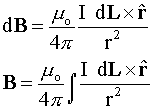For a very long wire carrying a current I, the field at a distance r from the wire is given by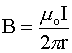Force between Parallel Conductors If the wires are each carrying currents I1 and I2, then setting up the B-field from one wire and calculating the force that B-field exerts on the current in the second wire gives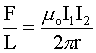Last modified on February 27, 2002